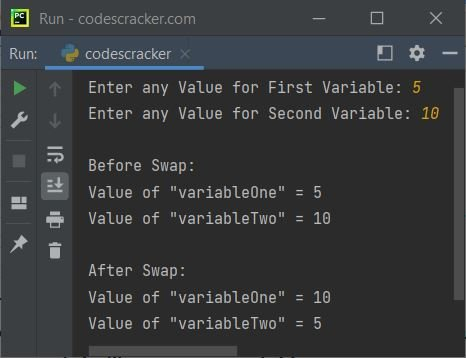# Python Program to Swap Two Variables

This article is created to cover a program in Python that swaps two variables. The program to swap two variables is created with and without using the third variable.

## Python: Swap Two Variables Using a Third Variable

The question is: write a Python program to swap two variables. The answer to this question is the program given below:

```print("Enter any Value for First Variable: ", end="")
variableOne = input()
print("Enter any Value for Second Variable: ", end="")
variableTwo = input()

print("\nBefore Swap:")
print("Value of \"variableOne\" =", variableOne)
print("Value of \"variableTwo\" =", variableTwo)

x = variableOne
variableOne = variableTwo
variableTwo = x

print("\nAfter Swap:")
print("Value of \"variableOne\" =", variableOne)
print("Value of \"variableTwo\" =", variableTwo)```

The snapshot given below shows the sample run of the above Python program with user input 5 and 10 as two values for the first and second variables, namely variableOne and variableTwo.The above example code takes two values as input from the user and then swaps them using a temporary variable. The print statements are used to display the values of the variables before and after the swap.

The input() function is used to take input from the user and store the values in the variables variableOne and variableTwo. Then, the values of these variables are printed using the print() function.

Next, the values of the two variables are swapped using a temporary variable x. The print() function is used again to display the values of the variables after the swap.

## Python: Swap Two Variables Without Using a Third Variable

To swap two variables in Python without using the third variable. Then replace the following statements from the previous program:

```x = variableOne
variableOne = variableTwo
variableTwo = x```

with a single statement given below:

`variableOne, variableTwo = variableTwo, variableOne`

That is, the value of variableTwo gets initialized to variableOne, whereas the value of variableOne gets initialized to variableTwo.

Python Online Test

« Previous Program Next Program »